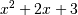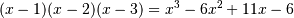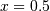# numpy.poly1d¶

class `numpy.``poly1d`(c_or_r, r=False, variable=None)[source]

A one-dimensional polynomial class.

A convenience class, used to encapsulate “natural” operations on polynomials so that said operations may take on their customary form in code (see Examples).

Parameters: c_or_r : array_like The polynomial’s coefficients, in decreasing powers, or if the value of the second parameter is True, the polynomial’s roots (values where the polynomial evaluates to 0). For example, `poly1d([1, 2, 3])` returns an object that represents, whereas `poly1d([1, 2, 3], True)` returns one that represents. r : bool, optional If True, c_or_r specifies the polynomial’s roots; the default is False. variable : str, optional Changes the variable used when printing p from x to `variable` (see Examples).

Examples

Construct the polynomial:

```>>> p = np.poly1d([1, 2, 3])
>>> print(np.poly1d(p))
2
1 x + 2 x + 3
```

Evaluate the polynomial at:

```>>> p(0.5)
4.25
```

Find the roots:

```>>> p.r
array([-1.+1.41421356j, -1.-1.41421356j])
>>> p(p.r)
array([ -4.44089210e-16+0.j,  -4.44089210e-16+0.j])
```

These numbers in the previous line represent (0, 0) to machine precision

Show the coefficients:

```>>> p.c
array([1, 2, 3])
```

Display the order (the leading zero-coefficients are removed):

```>>> p.order
2
```

Show the coefficient of the k-th power in the polynomial (which is equivalent to `p.c[-(i+1)]`):

```>>> p
2
```

Polynomials can be added, subtracted, multiplied, and divided (returns quotient and remainder):

```>>> p * p
poly1d([ 1,  4, 10, 12,  9])
```
```>>> (p**3 + 4) / p
(poly1d([  1.,   4.,  10.,  12.,   9.]), poly1d([ 4.]))
```

`asarray(p)` gives the coefficient array, so polynomials can be used in all functions that accept arrays:

```>>> p**2 # square of polynomial
poly1d([ 1,  4, 10, 12,  9])
```
```>>> np.square(p) # square of individual coefficients
array([1, 4, 9])
```

The variable used in the string representation of p can be modified, using the `variable` parameter:

```>>> p = np.poly1d([1,2,3], variable='z')
>>> print(p)
2
1 z + 2 z + 3
```

Construct a polynomial from its roots:

```>>> np.poly1d([1, 2], True)
poly1d([ 1, -3,  2])
```

This is the same polynomial as obtained by:

```>>> np.poly1d([1, -1]) * np.poly1d([1, -2])
poly1d([ 1, -3,  2])
```

Attributes

 `c` A copy of the polynomial coefficients `coef` A copy of the polynomial coefficients `coefficients` A copy of the polynomial coefficients `coeffs` A copy of the polynomial coefficients `o` The order or degree of the polynomial `order` The order or degree of the polynomial `r` The roots of the polynomial, where self(x) == 0 `roots` The roots of the polynomial, where self(x) == 0 `variable` The name of the polynomial variable

Methods

 `__call__`(val) `deriv`([m]) Return a derivative of this polynomial. `integ`([m, k]) Return an antiderivative (indefinite integral) of this polynomial.

Poly1d

numpy.poly1d.c